## 新手指南

### 枪械图鉴

1. AK47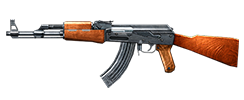伤害 50+20 射速 55+26 便携 32+23 精准 67+26 稳定 57+39 射程 85+35 穿甲 25+10 载弹 30+3

2. M4A1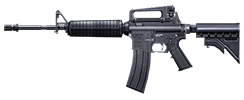伤害 40+20 射速 60+26 便携 31+23 精准 75+26 稳定 62+39 射程 90+35 穿甲 25+10 载弹 30+3

3. G36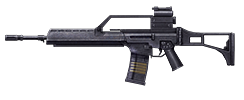伤害 48+20 射速 70+26 便携 30+23 精准 75+26 稳定 62+39 射程 87+35 穿甲 25+10 载弹 30+3

4. FAMAS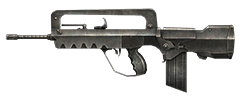伤害 42+20 射速 60+26 便携 31+23 精准 75+26 稳定 62+39 射程 90+35 穿甲 25+10 载弹 30+3

5. AUG伤害 45+20 射速 70+26 便携 32+23 精准 75+26 稳定 60+39 射程 90+35 穿甲 25+10 载弹 30+3

6. QBZ95伤害 50+20 射速 70+26 便携 30+23 精准 87+26 稳定 67+39 射程 85+35 穿甲 25+10 载弹 30+3

7. SCAR伤害 46+20 射速 70+26 便携 30+23 精准 75+26 稳定 62+39 射程 89+35 穿甲 25+10 载弹 30+3

8. 黄金AK47伤害 55+20 射速 55+26 便携 30+23 精准 67+26 稳定 57+39 射程 75+35 穿甲 25+10 载弹 35+3

9. 黄金M4A1伤害 45+20 射速 60+26 便携 30+23 精准 75+26 稳定 62+39 射程 85+35 穿甲 25+10 载弹 35+3

10. 水晶AK47伤害 57+20 射速 55+26 便携 28+23 精准 67+26 稳定 57+39 射程 75+35 穿甲 25+10 载弹 35+3

11. 水晶M4A1伤害 47+20 射速 60+26 便携 28+23 精准 75+26 稳定 62+39 射程 85+35 穿甲 25+10 载弹 35+3

1. MP5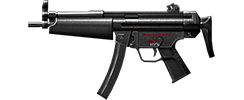伤害 42+20 射速 80+26 便携 25+23 精准 75+26 稳定 65+39 射程 28+35 穿甲 25+10 载弹 30+3

2. TMP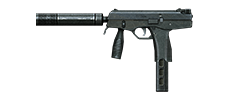伤害 40+20 射速 90+26 便携 23+23 精准 65+26 稳定 60+39 射程 25+35 穿甲 25+10 载弹 30+3

3. VECTOR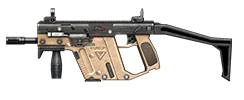伤害 50+20 射速 80+26 便携 23+23 精准 75+26 稳定 60+39 射程 20+35 穿甲 25+10 载弹 30+3

4. PP-2000

（暂无图示）

 伤害 45+20 射速 90+26 便携 23+23 精准 75+26 稳定 65+39 射程 25+35 穿甲 25+10 载弹 44+3

5. UMP-45

（暂无图示）

 伤害 50+20 射速 70+26 便携 25+23 精准 75+26 稳定 65+39 射程 20+35 穿甲 25+10 载弹 25+3

6. P90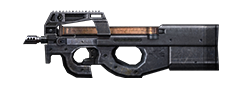伤害 45+20 射速 80+26 便携 25+23 精准 65+26 稳定 60+39 射程 25+35 穿甲 25+10 载弹 50+3

7. 黄金MP5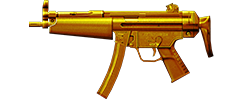伤害 47+20 射速 80+26 便携 25+23 精准 75+26 稳定 65+39 射程 28+35 穿甲 25+10 载弹 30+3

1. M249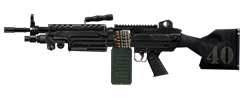伤害 45+20 射速 58+26 便携 45+23 精准 75+26 稳定 60+39 射程 90+35 穿甲 25+10 载弹 100+3

2. RPK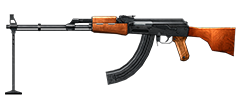伤害 47+20 射速 60+26 便携 38+23 精准 65+26 稳定 65+39 射程 88+35 穿甲 25+10 载弹 75+3

1. SPAS-12伤害 90+20 射速 6+26 便携 32+23 精准 32+26 稳定 55+39 射程 10+35 穿甲 25+10 载弹 8+2

2. M1014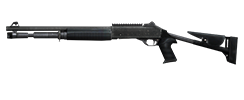伤害 75+20 射速 15+26 便携 30+23 精准 22+26 稳定 60+39 射程 2+35 穿甲 25+10 载弹 6+2

3. KSG

（暂无图示）

 伤害 90+20 射速 12+26 便携 28+23 精准 37+26 稳定 65+39 射程 10+35 穿甲 25+10 载弹 14+2

4. Striker-12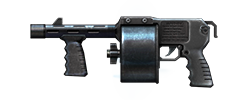伤害 75+20 射速 16+26 便携 30+23 精准 35+26 稳定 58+39 射程 7+35 穿甲 25+10 载弹 12+2

1. AWP伤害 100+20 射速 6+26 便携 40+23 精准 90+26 稳定 20+39 射程 100+35 穿甲 60+10 载弹 10+3

2. M82A1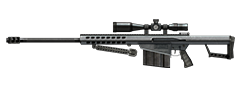伤害 130+20 射速 5+26 便携 42+23 精准 90+26 稳定 20+39 射程 100+35 穿甲 65+10 载弹 10+3

3. SVD伤害 100+20 射速 30+26 便携 35+23 精准 90+26 稳定 20+39 射程 100+35 穿甲 50+10 载弹 10+3

4. 黄金AWP伤害 130+20 射速 6+26 便携 40+23 精准 90+26 稳定 20+39 射程 100+35 穿甲 60+10 载弹 10+3

5. 水晶AWP伤害 150+20 射速 6+26 便携 38+23 精准 90+26 稳定 20+39 射程 100+35 穿甲 60+10 载弹 10+3

1. USP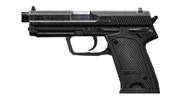伤害 40+20 射速 40+26 便携 23+23 精准 73+26 稳定 56+39 射程 20+35 穿甲 25+10 载弹 12+2

2. 沙漠之鹰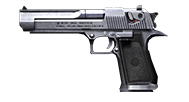伤害 60+20 射速 30+26 便携 23+23 精准 76+26 稳定 55+39 射程 30+35 穿甲 25+10 载弹 7+2

3. M9伤害 47+20 射速 40+26 便携 23+23 精准 75+26 稳定 58+39 射程 13+35 穿甲 25+10 载弹 15+2

4. QBZ92伤害 50+20 射速 35+26 便携 23+23 精准 75+26 稳定 58+39 射程 13+35 穿甲 25+10 载弹 15+2

5. 黄金沙漠之鹰伤害 65+20 射速 30+26 便携 23+23 精准 76+26 稳定 55+39 射程 32+35 穿甲 25+10 载弹 7+2

6. 水晶沙漠之鹰伤害 72+20 射速 30+26 便携 23+23 精准 76+26 稳定 55+39 射程 32+35 穿甲 25+10 载弹 7+2

1. 匕首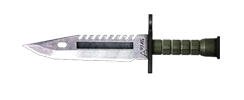伤害 75 攻速 10 范围 10

2. 战斧伤害 100 攻速 6 范围 13

3. 黄金匕首伤害 100 攻速 10 范围 16

4. 黄金战斧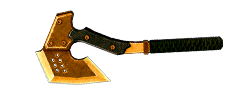伤害 120 攻速 6 范围 16

5. 手雷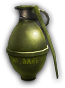威力 100 范围 10

6. 高爆手雷威力 120 范围 17